# Examples of FFTrees

## Examples of FFTs with FFTrees

This vignette illustrates how to construct fast-and-frugal trees (FFTs) for additional datasets included in the FFTrees package. (See Phillips, Neth, Woike, & Gaissmaier, 2017 for a comparison across 10 real-world datasets.)

### Mushrooms data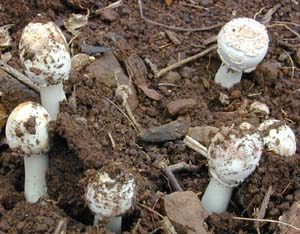The mushrooms dataset contains data about mushrooms (see ?mushrooms for details). The goal of our model is to predict which mushrooms are poisonous based on 22 cues ranging from the mushroom’s odor, color, etc.

Here are the first few rows and a subset of 10 potential predictors of the mushrooms data:

Table 1: Binary criterion variable poisonous and 10 potential predictors in the mushrooms data.
poisonous cshape csurface ccolor bruises odor vcolor ringnum ringtype sporepc population habitat
TRUE x s n t p w o p k s u
FALSE x s y t a w o p n n g
FALSE b s w t l w o p n n m
TRUE x y w t p w o p k s u
FALSE x s g f n w o e n a g
FALSE x y y t a w o p k n g

#### Creating FFTs

Let’s create some trees using FFTrees()! We’ll use the train.p = .50 argument to split the original data into a $$50$$% training set and a $$50$$% testing set:

# Create FFTs from the mushrooms data:
set.seed(1) # for replicability of the training / test data split

mushrooms.fft <- FFTrees(formula = poisonous ~.,
data = mushrooms,
train.p = .50,   # split data into 50:50 training/test subsets
main = "Mushrooms",
decision.labels = c("Safe", "Poison"))   

Here’s basic information about the best performing FFT (Tree #1):

# Print information about the best tree during training:
mushrooms.fft
#> Mushrooms
#> FFTrees
#> - Trees: 6 fast-and-frugal trees predicting poisonous
#> - Outcome costs: [hi = 0, mi = 1, fa = 1, cr = 0]
#>
#> FFT #1: Definition
#>  If odor != {f,s,y,p,c,m}, decide Safe.
#>  If sporepc = {h,w,r}, decide Poison, otherwise, decide Safe.
#>
#> FFT #1: Training Accuracy
#> Training data: N = 4,062, Pos (+) = 1,958 (48%)
#>
#> |          | True +   | True -   |   Totals:
#> |----------|----------|----------|
#> | Decide + | hi 1,683 | fa     0 |     1,683
#> | Decide - | mi   275 | cr 2,104 |     2,379
#> |----------|----------|----------|
#>   Totals:       1,958      2,104   N = 4,062
#>
#> acc  = 93.2%   ppv  = 100.0%   npv  = 88.4%
#> bacc = 93.0%   sens = 86.0%   spec = 100.0%
#>
#> FFT #1: Training Speed, Frugality, and Cost
#> mcu = 1.47,  pci = 0.93,  E(cost) = 0.068

#### Visualizing cue accuracies

Let’s look at the individual cue training accuracies with plot(fft, what = "cues"):

# Plot the cue accuracies of an FFTrees object:
plot(mushrooms.fft, what = "cues")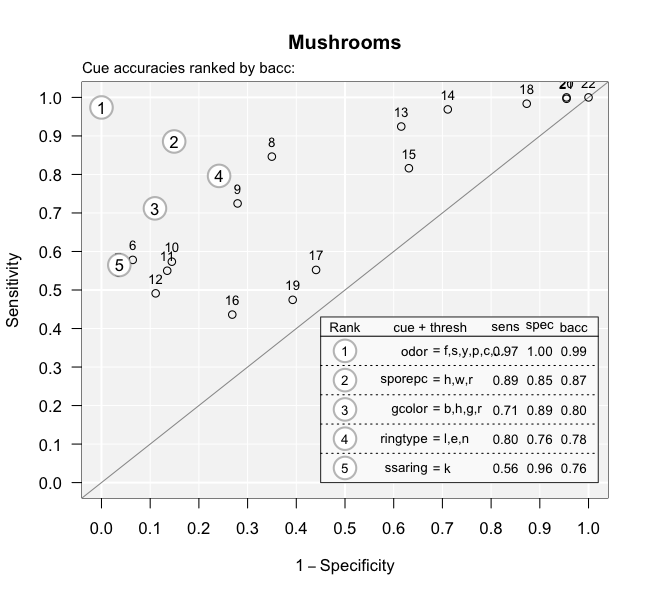It looks like the cues oder and sporepc are the best predictors. In fact, the single cue odor has a hit rate of $$97$$% and a false alarm rate of nearly $$0$$%! Based on this, we should expect the final trees to use just these cues.

#### Visualizing FFT performance

Now let’s plot the performance of the best training tree when applied to the test data:

# Plot the best FFT for the mushrooms test data:
plot(mushrooms.fft, data = "test")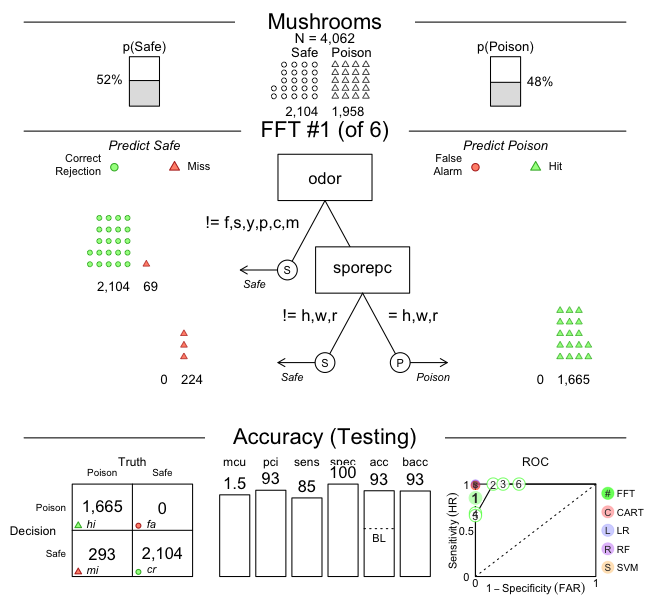Indeed, it looks like the best tree only uses the odor and sporepc cues. In our test dataset, the tree had a false alarm rate of $$0$$% ($$1 -$$ specificity), and a sensitivity (aka. hit rate) of $$85$$%. When considering the implications of our decisions, the fact that our FFT incurs many misses, but no false alarms, is problematic (as failing to detect poisonous mushrooms typically has more serious consequences than falsely classifying some as poisonous). To change this balance, we could increase the sensitivity weight parameter (e.g., setting sens.w = .67) and optimize the tree’s weighted accuracy wacc.

#### An alternative FFT

But let’s assume that a mushroom expert insists that we are using the wrong cues. According to her, the best predictors for poisonous mushrooms are ringtype and ringnum. Let’s build a set of trees with these cues and see how they perform relative to our initial tree:

# Create trees using only the ringtype and ringnum cues:
mushrooms.ring.fft <- FFTrees(formula = poisonous ~ ringtype + ringnum,
data = mushrooms,
train.p = .50,
main = "Mushrooms (ring only)",
decision.labels = c("Safe", "Poison"))

Here is the best training tree, when applied to predicting the cases in the test dataset:

# Plotting the best training FFT for test data:
plot(mushrooms.ring.fft, data = "test")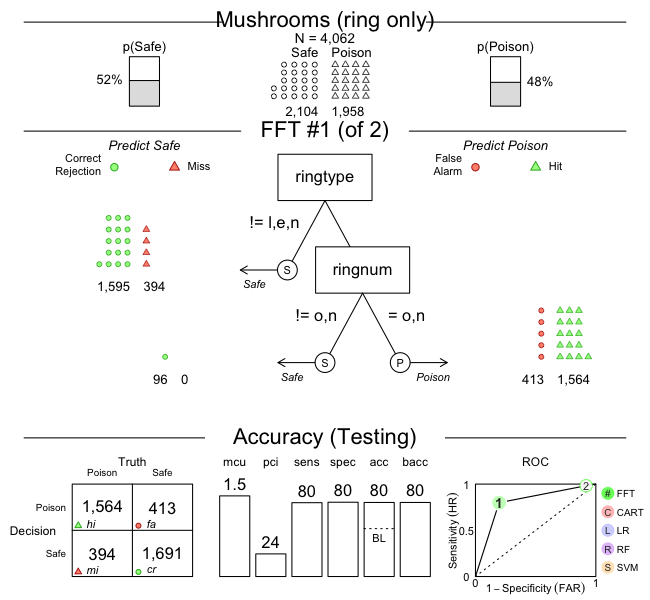As we can see, this tree (in mushrooms.ring.fft) has both a sensitivity and a specificity of around $$80$$%, but does not perform as well as our earlier one (in mushrooms.fft). This suggests that we should discard the expert’s advice and primarily rely on the odor and sporepc cues.

### Iris.v dataThe iris.v dataset contains data about 150 flowers (see ?iris.v). Our goal is to predict which flowers are of the class Virginica. In this example, we’ll create trees using the entire dataset (without splitting the available data into explicit training vs. test subsets):

# Create FFTrees object for iris data:
iris.fft <- FFTrees(formula = virginica ~.,
data = iris.v,
main = "Iris",
decision.labels = c("Not-V", "V"))

For summary information, we could print the FTrees object:

iris.fft

However, let’s take a look at the individual training cue accuracies instead…

#### Visualizing cue accuracies

We can plot the training cue accuracies during training by specifying what = "cues":

# Plot cue values:
plot(iris.fft, what = "cues")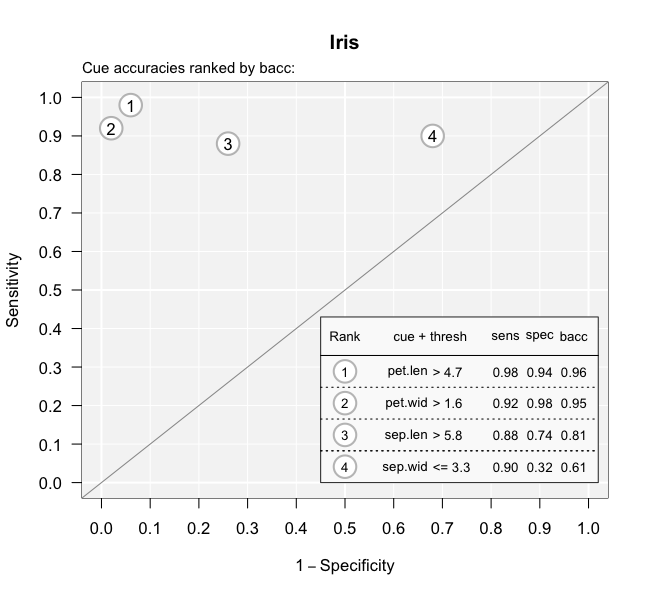It looks like the two cues pet.wid and pet.len are the best predictors for this dataset. Based on this, we should expect the final trees will likely use one or both of these cues.

#### Visualizing FFT performance

Now let’s examine the best tree:

# Plot best FFT (in training):
plot(iris.fft)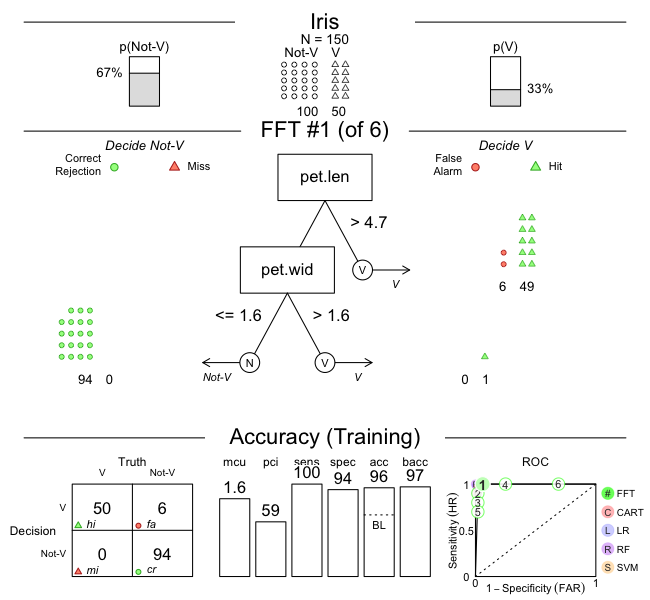Indeed, it turns out that the best tree only uses the pet.wid and pet.len cues. In our test data, the tree had a sensitivity of 100% and a specificity of 94%.

#### Alternative FFTs

Now, this tree did quite well, but what if someone wanted a tree with the lowest possible false alarm rate? If we look at the ROC plot in the bottom left corner of the plot above, we can see that Tree #2 has a specificity close to 100%. Let’s look at that tree:

# Plot FFT #2 in iris FFTrees:
plot(iris.fft, tree = 2)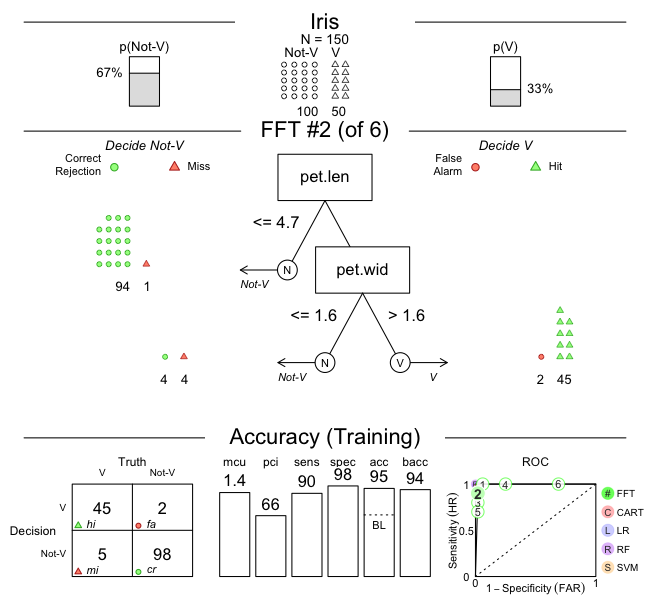As we can see, this tree does indeed have a higher specificity (of 98%). However, this increase comes at a cost of a lower sensitivity (of 90%).

Such trade-offs between measures are typical when fitting and predicting real-world data. Importantly, FFTs (and the FFTrees package) help us to render such trade-offs more transparent.

### Titanic data

For example FFTs that predict people’s survival of the Titanic disaster (using the titanic data), see the Visualizing FFTs with plot() vignette.

## Vignettes

Here is a complete list of the vignettes available in the FFTrees package:

Vignette Description
Main guide: FFTrees overview An overview of the FFTrees package
1 Tutorial: FFTs for heart disease An example of using FFTrees() to model heart disease diagnosis
2 Accuracy statistics Definitions of accuracy statistics used throughout the package
3 Creating FFTs with FFTrees() Details on the main function FFTrees()
4 Manually specifying FFTs How to directly create FFTs with my.tree without using the built-in algorithms
5 Visualizing FFTs with plot() Plotting FFTrees objects, from full trees to icon arrays
6 Examples of FFTs Examples of FFTs from different datasets contained in the package